﻿

### The influence of minimal subgroups on saturated fusion systems

#### Abstract

Let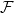$\cal F$ be a saturated fusion system over a \py group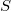$S$. In this paper, we investigate the influence of the minimal subgroups in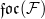$\mathfrak{foc}(\mathcal{F})$, the focal subgroup of$\cal F$. Our main result is that if for each cyclic subgroup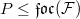$P\leq \mathfrak{foc}(\mathcal{F})$ of order$p$ (of order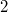$2$ and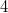$4$ if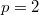$p=2$) and each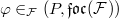$\varphi\in \sHom_{\cal F}(P,\mathfrak{foc}(\mathcal{F}))$,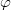$\varphi$ extends to an automorphism of$S$, then$S$ is normal in$\cal F$. We also give several applications of this result

DOI Code: 10.1285/i15900932v36suppl1p91

Keywords: saturated fusion system; focal subgroup; resistant group

Full Text: PDF